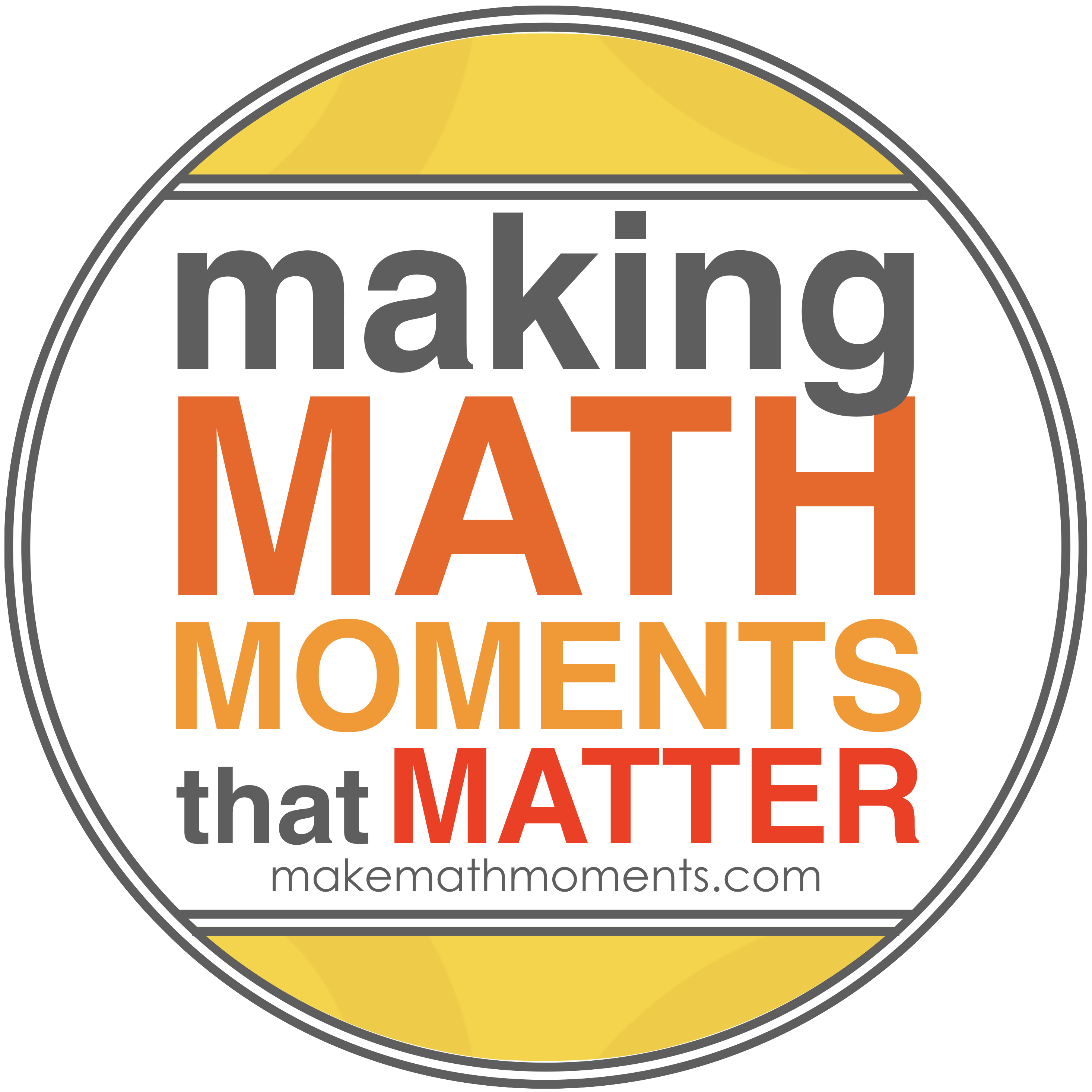# MPM2D

Course Description:

This course enables students to broaden their understanding of relationships and extend their

problem-solving and algebraic skills through investigation, the effective use of technology, and

abstract reasoning. Students will explore quadratic relations and their applications; solve and

apply linear systems; verify properties of geometric figures using analytic geometry; and investigate the trigonometry of right and acute triangles. Students will reason mathematically and

communicate their thinking as they solve multi-step problems.

Overall Learning Expectations:

By the end of this course, students will:

• determine the basic properties of quadratic relations;
• relate transformations of the graph of y = x2 to the algebraic representation

y = a(x – h)2 + k;

• solve quadratic equations and interpret the solutions with respect to the corresponding

relations;

• solve problems involving quadratic relations.
• model and solve problems involving the intersection of two straight lines;
• solve problems using analytic geometry involving properties of lines and line segments;
• verify geometric properties of triangles and quadrilaterals, using analytic geometry.
• use their knowledge of ratio and proportion to investigate similar triangles and solve

problems related to similarity;

• solve problems involving right triangles, using the primary trigonometric ratios and the

Pythagorean theorem;

• solve problems involving acute triangles, using the sine law and the cosine law.

Evaluation:

Term Work 70%

Knowledge / Understanding 35 %

Inquiry / Thinking 15 %

Communication 15 %

Application / Making Connections 35 %

 Application Communication Knowledge Thinking / Problem Solving Achievement checks   Assignments   Tests Appropriate use of mathematical language and symbols on quizzes and tests   Achievement checks Quizzes   Tests TIPS problems – End of unit problems

Summative assessment activities during the course will be comprised of a variety of methods and strategies.  (eg.  Assignments, projects, tests, journals, performances, conferences, etc.)

Final Evaluation 30%

The final evaluation will consist of, but is not limited to, a final examination, written during the examination schedule.

TIPS for success:

Students are expected to follow these rules so that mathematics education can be successful.

a) ATTENDANCE – Students should be present for all classes. If an absence is necessary, then students should obtain notes, worksheets, and homework from the teacher. The missed work should be completed; the student will be responsible for the material on the next quiz, assignment, and test.

b) HOMEWORK – All homework must be completed. If a student is having difficulty then still, every attempt should be made to try each homework question.

c) EXTRA HELP – Students should talk to the teacher if they need extra help. Sometimes the problem is big, and sometimes it is small. It is always better to clear up small problems before they become big problems.

Learning Materials Required:

•       Textbook: Book Number: __________
•       Notebook – 3 ring binder with lined and graph paper
•       Pencil, eraser, ruler
•       Scientific calculator (TI 83/84 preferred but not mandatory)
•       Student Handbook## CONTACT ME